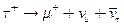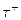Chapter 33, Problem 17PE

Chapter
Section
Textbook Problem

The decay mode of the positive tau is(a) What energy is released?(b) Verify that charge and lepton family numbers are conserved.(c) Theis the antiparticle of theVerify that all the decay products of theare the antiparticles of those in the decay of thegiven in the text.

To determine

(a)

The energy released in the decay of positive tau particle given by τ+μ++νμ+ν¯τ

Explanation

Given:

The decay mode of negative muon is given by, τ+μ++νμ+ν¯τ

Formula used:

Energy released = Rest energy of initial particle − sum of energies of decay products

Calculation:

In this problem the positive tau decays by τ+μ++νμ+ν¯τ

Rest energy of τ+ particle =1777 MeV

Rest energy of μ+ particle =105

To determine

(b)

The conservation of charge and lepton family numbers.

To determine

(c)

Whether all the decay products of τ+ particle are the antiparticles of those in the decay of

τ

Still sussing out bartleby?

Check out a sample textbook solution.

See a sample solution

The Solution to Your Study Problems

Bartleby provides explanations to thousands of textbook problems written by our experts, many with advanced degrees!

Get Started